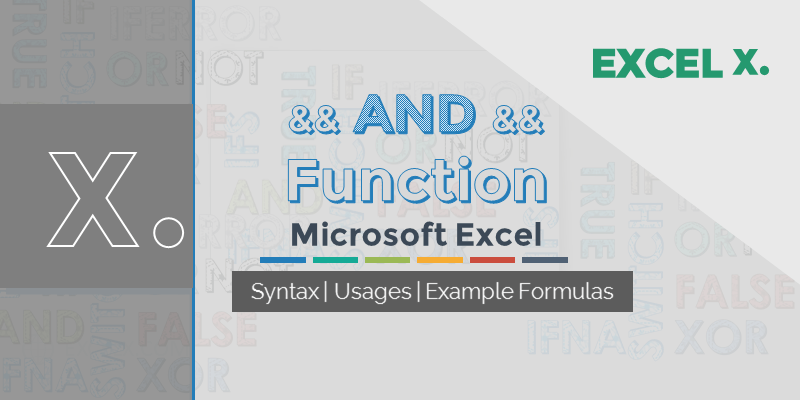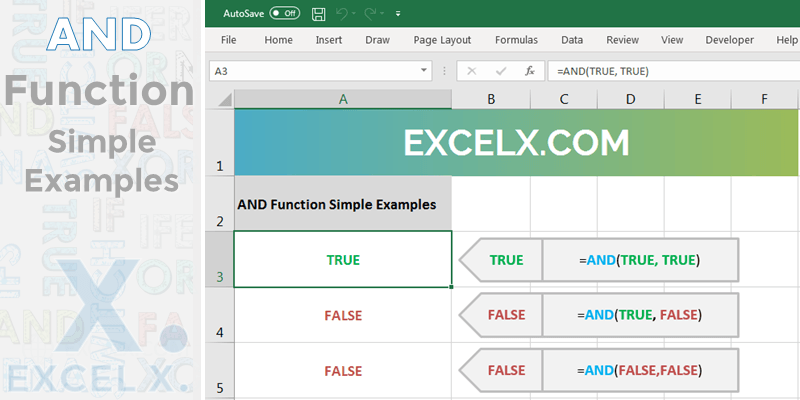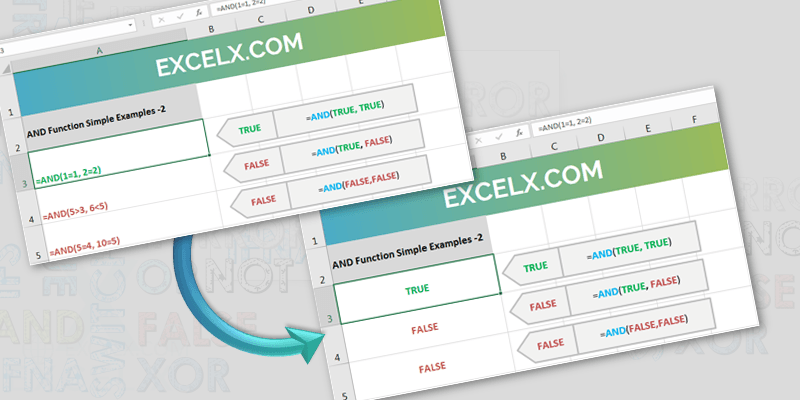AND function is helps to check if all conditions are True. AND function returns TRUE if all the expressions passed as its arguments are true, it return FALSE if any of the expressions is False.

## Syntax of AND Function in Excel

Here is the syntax of Excel AND Function. Syntax of the AND function contains AND Keyword and the logical expressions.+

=AND(Logical expression 1[, Logical Expression 2,…., Logical Expression 255])

We must provide at least one logical expression as function argument.AND Function in Microsoft Excel can check up to 255 logical expressions. AND function will return TRUE or FALSE as a result for the given arguments.

### Simple Example of AND Function in Excel

Here are the simple example using AND function in Excel. You observe the arguments of each AND formula and it’s result as Boolean TRUE or FALSE. Here is the screen-shot of Simple Excel Formulas with AND Function and it return values.Example – 1: The following example will check the Two condition and return True as both the conditions are TRUE. You can copy the below formula and enter in Range ‘A3’ in the worksheet. It will return TRUE as result.

=AND(TRUE, TRUE)

Example – 2: The following example will check the Two condition and return FALSE as one of the condition is FALSE. You can copy the below formula and enter in Range ‘A4’ in the worksheet. It will return FALSE as result.

=AND(TRUE, FALSE)

Example – 3: The following example will check the Two condition and return FALSE as both of the condition are FALSE. You can copy the below formula and enter in Range ‘A5’ in the worksheet. It will return FALSE as result.

=AND(FALSE, FALSE)

### Example of AND Function in Excel